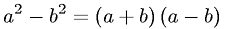Equations > Algebra > Polynomials > Difference of Squares Factorization

### Difference of Squares FactorizationLatex Code:

MathML Code:

 ${a}^{2}-{b}^{2}=\left(a+b\right)\left(a-b\right)$

MathType 5.0: